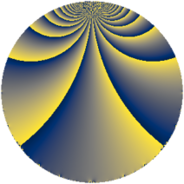# Properties

 Label 60.3.lLevel $60$ Weight $3$ Character orbit 60.l Rep. character $\chi_{60}(23,\cdot)$ Character field $\Q(\zeta_{4})$ Dimension $40$ Newform subspaces $1$ Sturm bound $36$ Trace bound $0$

# Learn more

## Defining parameters

 Level: $$N$$ $$=$$ $$60 = 2^{2} \cdot 3 \cdot 5$$ Weight: $$k$$ $$=$$ $$3$$ Character orbit: $$[\chi]$$ $$=$$ 60.l (of order $$4$$ and degree $$2$$) Character conductor: $$\operatorname{cond}(\chi)$$ $$=$$ $$60$$ Character field: $$\Q(i)$$ Newform subspaces: $$1$$ Sturm bound: $$36$$ Trace bound: $$0$$

## Dimensions

The following table gives the dimensions of various subspaces of $$M_{3}(60, [\chi])$$.

Total New Old
Modular forms 56 56 0
Cusp forms 40 40 0
Eisenstein series 16 16 0

## Trace form

 $$40q - 4q^{6} + O(q^{10})$$ $$40q - 4q^{6} - 12q^{10} - 20q^{12} - 8q^{13} - 36q^{16} - 24q^{18} - 24q^{21} - 76q^{22} - 8q^{25} - 84q^{28} + 68q^{30} - 40q^{33} + 172q^{36} - 40q^{37} + 104q^{40} + 236q^{42} - 104q^{45} + 240q^{46} + 196q^{48} + 304q^{52} - 72q^{57} + 180q^{58} - 284q^{60} + 48q^{61} - 552q^{66} - 372q^{70} - 600q^{72} + 104q^{73} - 736q^{76} - 408q^{78} + 72q^{81} - 720q^{82} + 216q^{85} - 580q^{88} + 528q^{90} + 368q^{93} + 884q^{96} + 72q^{97} + O(q^{100})$$

## Decomposition of $$S_{3}^{\mathrm{new}}(60, [\chi])$$ into newform subspaces

Label Dim. $$A$$ Field CM Traces $q$-expansion
$$a_2$$ $$a_3$$ $$a_5$$ $$a_7$$
60.3.l.a $$40$$ $$1.635$$ None $$0$$ $$0$$ $$0$$ $$0$$